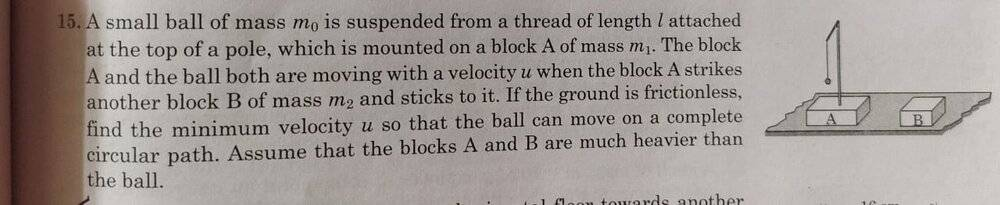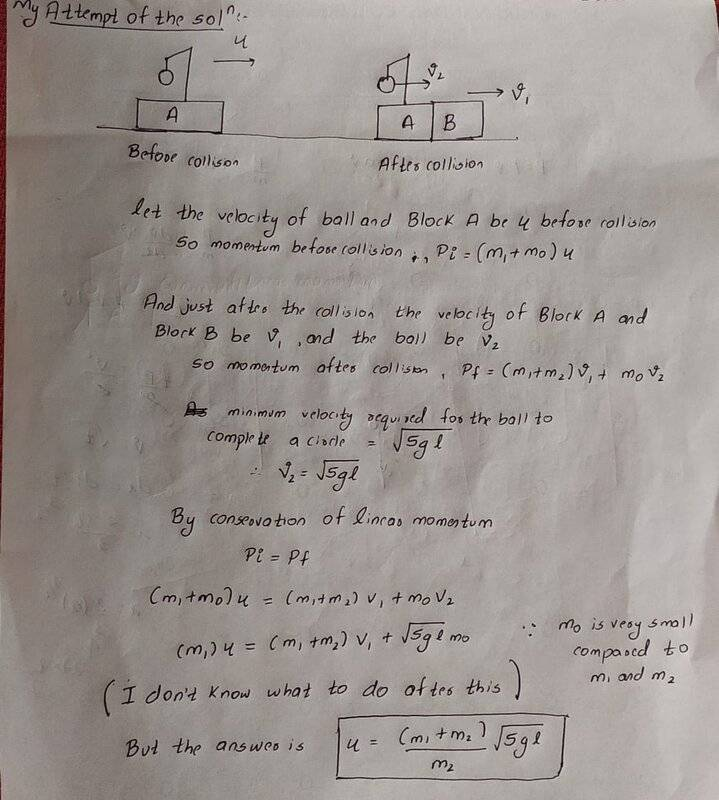# Momentum conservation question

Homework Statement:
statement and diagram are in the attached pic
Relevant Equations:
.•Delta2

haruspex
Homework Helper
Gold Member
2020 Award
Per forum rules, please quote any standard equations you suppose to be relevant and, more importantly, some attempt.

•PCAPS
Per forum rules, please quote any standard equations you suppose to be relevant and, more importantly, some attempt.•Delta2
haruspex
Homework Helper
Gold Member
2020 Award
During the brief period of impact, what horizontal force does the ball exert on its support?

During the brief period of impact, what horizontal force does the ball exert on its support?
tension?

•Steve4Physics
haruspex
Homework Helper
Gold Member
2020 Award
tension?
During the (assumed very brief) impact, the string will still be nearly vertical.

•PCAPS
During the (assumed very brief) impact, the string will still be nearly vertical.
Im still not able to get the solutionSteve4Physics
Homework Helper
Gold Member
Homework Statement:: statement and diagram are in the attached pic
Relevant Equations:: .

View attachment 277188
A few things to think about...

You have ignored Newton’s 1st law! Just before the collision, the ball has (horizontal) velocity u. What is its velocity just after the collision (and why)? (Consider @haruspex's replies.)

You are told the blocks are much heavier than the ball. When calculating the velocity of [A and B stuck together] using conservation of momentum, you can simply ignore the mass of the ball.

You have used ##v_{min} = \sqrt{5gh}##. This is not usually a ‘standard formula’ but, if you are allowed to use it without derivation, OK.

The above formula gives ##v_{min}## in a frame of reference in which the centre of the circular rotation is stationary. This is not the lab’ frame. It may help if you imagine you are an observer sitting on block A. Note that ##v_{min}## is a velocity relative to you (just after impact).

[EDIT: Minor edits improve wording.]

Last edited:
•Lnewqban and PCAPS
A few things to think about...

You have ignored Newton’s 1st law! Just before the collision, the ball has (horizontal) velocity u. What is its velocity just after the collision (and why)? (Consider @haruspex's replies.)

You are told the blocks are much heavier than the ball. When calculating the velocity of [A and B stuck together] using conservation of momentum, you can simply ignore the mass of the ball.

You have used ##v_{min} = \sqrt{5gh}##. This is not usually a ‘standard formula’ but, if you are allowed to use it without derivation, OK.

The above formula gives ##v_{min}## in a frame of reference in which the centre of the circular rotation is stationary. This is not the lab’ frame. It may help if you imagine you are an observer sitting on block A. Note that ##v_{min}## is a velocity relative to you (just after impact).

[EDIT: Minor edits improve wording.]
I think I got the answer, can u validate my method?

#### Attachments

•Steve4Physics
Steve4Physics
Homework Helper
Gold Member
I think I got the answer, can u validate my method?
Looks good. Well done!

EDIT: As a further point of clarification, since the string is vertical, there is no horizontal force acting on the ball . Therefore there is no horizontal acceleration and the ball's velocity remains u (until the ball starts moving upwards after the collision).

•PCAPS
Looks good. Well done!
thankyouDuring the (assumed very brief) impact, the string will still be nearly vertical.
thankyou too for providing hints instead of plain answer, I am happy that I have done it myself .It was bothering me from the morning.

•Lnewqban
PeroK
Homework Helper
Gold Member
2020 Award
thankyouThe first equation you wrote is not quite right. You should have: $$m_1u + m_0u = (m_1 + m_2)v + m_0u$$ The mass ##m_0## disappears from the equation at this point. You use ##m_0 << m_1 + m_2## subsequently, by ignoring any change in the motion of ##m_1 +m_2## as ##m_0## executes its circuit.

•PCAPS
The first equation you wrote is not quite right. You should have: $$m_1u + m_0u = (m_1 + m_2)v + m_0u$$ The mass ##m_0## disappears from the equation at this point. You use ##m_0 << m_1 + m_2## subsequently, by ignoring any change in the motion of ##m_1 +m_2## as ##m_0## executes its circuit.
thanks for pointing that out, is the latter part correct?

PeroK
•